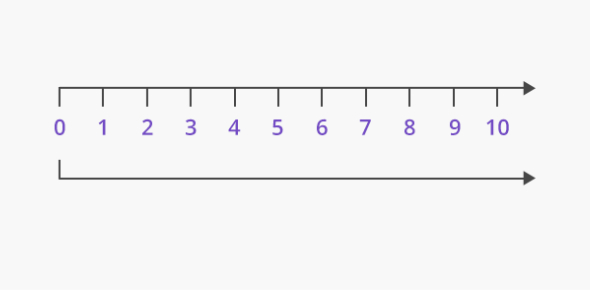# Whole Numbers MCQ Math Quiz!

10 Questions | Total Attempts: 944Settings.

• 1.
The successor of 200.
• A.

201

• B.

202

• C.

199

• D.

200

• 2.
The numbers starts from 0 are:
• A.

Wholenumbers

• B.

Natural numbers

• C.

Integers

• D.

Rational numbers

• 3.
The product of 23 and 12:
• A.

276

• B.

236

• C.

245

• D.

298

• 4.
The sum of 132,98,2:
• A.

13200

• B.

19800

• C.

14200

• D.

13250

• 5.
• A.

A+b is a wholenumber

• B.

A-b is a whole number

• C.

Axb is a whole number

• D.

A/b is a whole number

• 6.
The predecessor of whole number 0:
• A.

None

• B.

10

• C.

1

• D.

0

• 7.
0+1=?
• A.

0

• B.

10

• C.

None

• D.

1

• 8.
0x1=?
• A.

1

• B.

0

• C.

None

• D.

10

• 9.
0/1=?
• A.

0

• B.

1

• C.

Meaningless

• D.

None of the above

• 10.
All the whole numbers are natural numbers.
• A.

True

• B.

False

Related TopicsBack to top